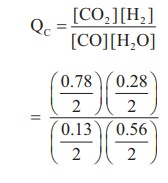Home | | Chemistry 11th std | Evaluate Yourself - Chemistry: Physical and Chemical Equilibrium

# Evaluate Yourself - Chemistry: Physical and Chemical Equilibrium

Chemistry : Physical and Chemical Equilibrium - Evaluate Yourself - Chemistry: Physical and Chemical Equilibrium

## Evaluate Yourself

1) Consider the following reaction

Fe3+(aq) + SCN(aq)  [Fe(SCN)]2+(aq)

A solution is made with initial Fe3+, SCN- concentration of 1 x 10-3M and 8 x 10-4 M respectively. At equilibrium [Fe(SCN)]2+ concentration is 2 x 10-4M. Calculate the value of equilibrium constant.

### Solution:2) The atmospheric oxidation of NO

2NO(g) + O2(g)  2NO2(g)

was studied with initial pressure of 1 atm of NO and 1 atm of O2. At equilibrium, partial pressure of oxygen is 0.52 atm calculate Kp of the reaction.

### Solution:3) The following water gas shift reaction is an important industrial process for the production of hydrogen gas.

CO(g) + H2O(g)  CO2(g) + H2(g)

At a given temperature Kp = 2.7. If 0.13 mol of CO, 0.56 mol of water, 0.78 mol of CO2 and and 0.28 mol of H2 are introduced into a 2 L flask, and find out in which direction must the reaction proceed to reach equilibrium

### Solution:

CO(g) + H2O(g)  CO2 (g) + H2(g)

Given KP = 2.7

[CO] = 0.13, [H2O] = 0.56

[CO2] = 0.78 ; [H2] = 0.28

V = 2L

KP = KC (RT)

2.7 = KC (RT)º

KC = 2.7Q = 3

Q > KC, Hence the reaction proceed in the reverse direction.

4) 1 mol of PCl5, kept in a closed container of volume 1 dm3 and was allowed to attain equilibrium at 423 K. Calculate the equilibrium composition of reaction mixture. (The Kc value for PCl5 dissociation at 423 K is 2)

### Solution:5) The equilibrium constant for the following reaction is 0.15 at 298 K and 1 atm pressure.

N2O4(g)  2NO2(g);

ΔHºf = 57.32 KJmol-1

The reaction conditions are altered as follows.

a) The reaction temperature is altered to 100o C keeping the pressure at 1 atm,

Calculate the equilibrium constant.

### Solution:

N2O4(g)  2NO2(g)

T1 = 298 K

KP1 = 0.15

T2 = 100º C = 100 + 273 = 373 K ;

KP2= ?KP2=104.7x0.15

KP2=15.705

Tags : Solved Example Problems , 11th Chemistry : UNIT 8 : Physical and Chemical Equilibrium
Study Material, Lecturing Notes, Assignment, Reference, Wiki description explanation, brief detail
11th Chemistry : UNIT 8 : Physical and Chemical Equilibrium : Evaluate Yourself - Chemistry: Physical and Chemical Equilibrium | Solved Example Problems